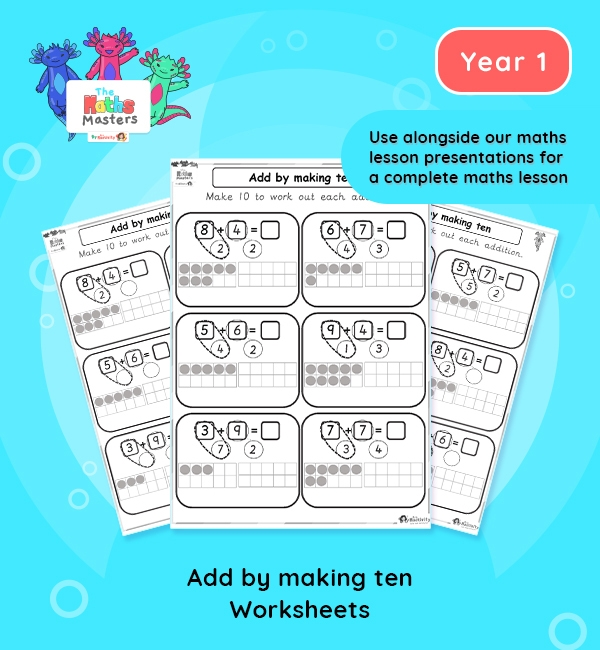# Year 1 | Adding by Making 10 Worksheets## Year 1 addition and subtraction worksheets

Aligned with the maths mastery approach, these Year 1 | Adding by Making 10 Worksheets are designed to be used alongside our Year 1 | Adding by Making 10 Lesson Presentation for a complete maths lesson. The maths worksheets requires children to use their knowledge of number bonds to 10  to complete some simple additions using ten frames. The worksheet can also be cut up and used individually in children’s books to demonstrate knowledge and progression, without the need for a whole worksheet.

Topic: Addition and subtraction to 20

This lesson practises adding numbers to 10 by making ten and then adding the remaining ones.

You may wish to spend one session recapping making ten. Then move onto a second session on making ten to help with adding numbers to 20.

Small Steps: Add by making 10.

NC Links: Read, write and interpret mathematical statements involving addition, subtraction and equals signs. *Add and subtract one-digit and two-digit numbers to 20, including zero. *Solve one step problems that involve addition and subtraction using concrete objects and pictorial representations , and missing number problems.

TAF Statements: Working towards: Add and subtract one digit number explaining their method verbally, in pictures, or using apparatus. Recall at least four of the six number bonds for 10 and reason about associated facts.

Working At: Recall all the number bonds to and within 10 and use these to reason with and calculate bonds to and within 20, recognising other associated additive relationships.

Greater Depth – Use reasoning about number and relationships to solve more complex problems and explain their thinking.

Ready-to-progress criteria:  1AS-1 Compose numbers to 10 from 2 parts.

1AS-2 Read, write and interpret equations containing addition, subtraction and equals symbols and relate additive expressions and equations to real-life contexts.

Previous experience: Devise and record number stories, using pictures, numbers and symbols.

## Recently Viewed